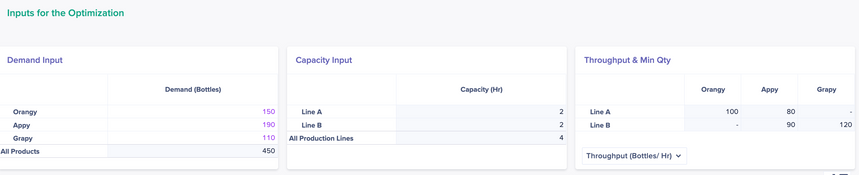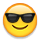# Demystify non-linear optimization: Episode 1## Prologue

The Anaplan community has been facing more and more interesting optimization problem statements every day while they solve complex business problems. Business challenges often involve finding optimal assignments such as sales reps to customers, demand to production capacity, or workers to shifts – all while obeying constraints.

However, sometimes the heroes face unprecedented non-linear problem statements which pose challenges to the linear optimization engine of Anaplan. Optimizer is Anaplan's implementation of a linear programming solver. Linear programming is a mathematical approach to finding ideal solutions to complex problems. It requires that we state our objective and constraints as linear expressions like a*X + b*Y - c*Z >= d. This requirement can force us to get creative to re-state the problem in a form that works. In this series, we will try to uncover some of the commonly faced non-linear optimization and the possible solutions to the same.

If you are new to optimization/ linear programming,  I suggest reading this Optimizer Toolbox Article by Samuel Wong first.

## Episode 1 (IF...THEN logic)

You have been approached by one of the new players in the beverage industry. They want to leverage Anaplan for production planning. They have 3 products: Orangy, Appy, and Grapy. They have 2 manufacturing lines, Line A and Line B, where the products are produced and packed. Line A can produce Orangy and Appy while Line B can produce Appy and Grapy. Throughput (quantity per hour) is provided in the table below:

 Line Product Throughput (bottles/ hour) Line A Orangy 100 Line A Appy 80 Line B Appy 90 Line B Grapy 120

Because of the nature of the product, the manufacturer does not want to produce more than the demand. On a certain week, the demand for the product was as follows: Orangy 150 bottles; Appy 190 bottles; and, Grapy 110 bottles.

Their machines have a particular setting for each of the products; for each line and product combination, a minimum quantity needs to get produced. If the demand is less than this minimum quantity, then they are ready to forego those sales.

 Line Product Minimum Quantity (bottles) Line A Orangy 100 Line A Appy 80 Line B Appy 90 Line B Grapy 120

Each line has 2 hours of capacity available for the week.

• Line B can only produce if both Appy and Grapy get produced. If one of them does not get produced, line B can not produce only one of the products.

They want to maximize production given the operational constraints.

The first step to solving an optimization problem is to identify the necessary components of the solution. Each of the business problem statements needs to be converted into equivalent mathematical formulations. This is the most important step of all. In this phase, you will also be able to identify the possible non-linearities hidden in the innocuous business problems.

## Analysis

Basic construct:

• We have 3 products and 2 manufacturing lines. Create products and manufacturing lines as Anaplan lists.
• Demand input:  a module with product dimension
• Capacity input: a module with manufacturing lines as dimensions
• The throughput matrix is given by product and lines. This can be captured in a module with dimensions of product and line. This also gives us the compatibility matrix (which product can get produced on which line).
• We also have a minimum production quantity for each product. This can be captured in the same module as above.

Let’s identify the components which are non-linear in nature:

• If a line produces a product, then a minimum quantity needs to get produced. This means that if there is no production, the minimum quantity rule does not apply. However, if there is production, it needs to be greater than the minimum quantity. Before we solve the problem, we do not know whether the line is going to produce a product or not. Hence, this needs to get determined at the run time of the optimizer as a variable.
• Line B can only produce if both Appy and Grapy get produced. Again, we do not know whether both will get produced or not before we do the optimization. Hence, this needs to get determined at the run time of the optimizer.

Finally, the objective is to maximize production (i.e., minimize demand shortage). We can treat this as a maximization problem of overall production or minimization of demand shortage (the second one followed here).

## Solution outline

The setup for inputs for the optimization can be done as depicted in the picture below:Once, the setup is done, we will create 3 modules to house the variables, constraints, and objectives of the optimization problem. An optimization problem should be attempted in steps. The first step here is to set up product and line production quantity and hours based on the throughput.
Below is the construct for the same:

```VAR01: Production Qty (Dimension: Product, Production Lines) - Integer variable (as the unit of measure is bottle), lower limit 0
VAR02: Production Hours (Dimension: Product, Production Lines) - Real variable, lower limit 0
VAR03: Unmet Demand (Dimension: Product) - Integer variable, lower limit 0```
```CONS01: Hours Quantity Match (Dimension: Product, Production Lines) - 'OPT01: Episode 1 Variables'.'VAR01: Production Qty' - 'OPT01: Episode 1 Variables'.'VAR02: Production Hours' * 'Throughput by Line & Product'.'Throughput (Bottles/ Hr)' = 0

CONS02: Only Feasible Combinations (Dimension: Product, Production Lines) - 'OPT01: Episode 1 Variables'.'VAR02: Production Hours' <= 'Throughput by Line & Product'.Feasible? [Feasibility is a derived line item and a large number if the throughput >0]

CONS03: Demand Constraint (Dimension: Product) - 'OPT01: Episode 1 Variables'.'VAR01: Production Qty' + 'OPT01: Episode 1 Variables'.'VAR03: Unmet Demand' = Demand by Product.'Demand (Bottles)'

CONS04: Capacity Constraint (Dimension: Production Lines) - 'OPT01: Episode 1 Variables'.'VAR02: Production Hours' <= Capacity by Lines.'Capacity (Hr)'```

The next step is to identify whether a product is getting produced on a line or not. This will be a dependent variable based on either VAR01 or VAR02. We will create a binary variable (x) to assign a value of 1 if production>0 and a value of 0 if production=0. In order to do this, we need to create an input of large number (also known as BigM). Assign a very large value to this. Below is the construct to assign the binary constraint.

• Equation 1: VAR01 - x * BigM <=0
• Equation 2: VAR01 - x>=0

If VAR01 is 10, then x has to assume a value of 1 in order to satisfy the first equation. the second equation gets satisfied automatically. If VAR01 is 0, then x has to assume a value of 0 in order to satisfy the second equation. The construct hence becomes:

```VAR04: BIN - Production Indicator - Binary variable
CONS05: BIN Production Indicator 1 - 'OPT01: Episode 1 Variables'.'VAR01: Production Qty' - 'OPT01: Episode 1 Variables'.'VAR04: BIN - Production Indicator' * 'SYS01: Global Lookup'.BigM <= 0

CONS06: BIN Production Indicator 2 - 'OPT01: Episode 1 Variables'.'VAR01: Production Qty' - 'OPT01: Episode 1 Variables'.'VAR04: BIN - Production Indicator' >= 0
(All dimensionalized by product and production lines)```

Next, we need to identify whether Line B is producing both Appy and Grapy, and then match the production indicator with this new variable. We already have a binary variable (VAR04) denoting whether Appy and Grapy are getting produced on line B or not. In this step, we need to multiply two instances of the same variable (Appy-Line B and Grapy-Lineto get one indicator. In order to do this, we will use two line items in a system module to capture the two products (which will be used for lookup). In essence, this is the multiplication of two binary variables. If x and y are binary variables and z is the product of x and y, then we can linearize the same using the following equations (validate the equations by substituting the values of x,y,z):

• Equation 1: z <= x
• Equation 2: z <= y
• Equation 3: z - x - y >= -1

Variables and constraints in Anaplan will be:

```VAR05: Product Group Production - Binary variable

CONS07: Product Group Production Indicator 1 - 'OPT01: Episode 1 Variables'.'VAR05: Product Group Production' - 'OPT01: Episode 1 Variables'.'VAR04: BIN - Production Indicator'[LOOKUP: 'SYS01: Global Lookup'.'Product 1'] <= 0

CONS08: Product Group Production Indicator 2 - 'OPT01: Episode 1 Variables'.'VAR05: Product Group Production' - 'OPT01: Episode 1 Variables'.'VAR04: BIN - Production Indicator'[LOOKUP: 'SYS01: Global Lookup'.'Product 2'] <= 0

CONS09: Product Group Production Indicator 3 - 'OPT01: Episode 1 Variables'.'VAR05: Product Group Production' - 'OPT01: Episode 1 Variables'.'VAR04: BIN - Production Indicator'[LOOKUP: 'SYS01: Global Lookup'.'Product 1'] - 'OPT01: Episode 1 Variables'.'VAR04: BIN - Production Indicator'[LOOKUP: 'SYS01: Global Lookup'.'Product 2'] >= -1

CONS10: Line B Production Condition - NOT 'SYS02: Production Group Constraint'.Product Group Consideration? OR 'OPT01: Episode 1 Variables'.'VAR04: BIN - Production Indicator' - 'OPT01: Episode 1 Variables'.'VAR05: Product Group Production' <= 0
(CONS10 is by product and production lines, rest by production line)```

For CONS10, we have used a system module to determine whether the constraint is to be applied to a line or not. This can be controlled by end-users. Once the setup is done, the objective function can either be maximizing VAR01 or minimizing VAR03. The modules used and a possible dashboard is attached. Change the input numbers or constraints, and see how it affects the results.

Sample Model Link: Optimization - Episode 1

Did you learn something new and exciting? Do you have some problem statements that you have encountered in past? Would love to hear from you, let us know in the comments below.

Tagged: